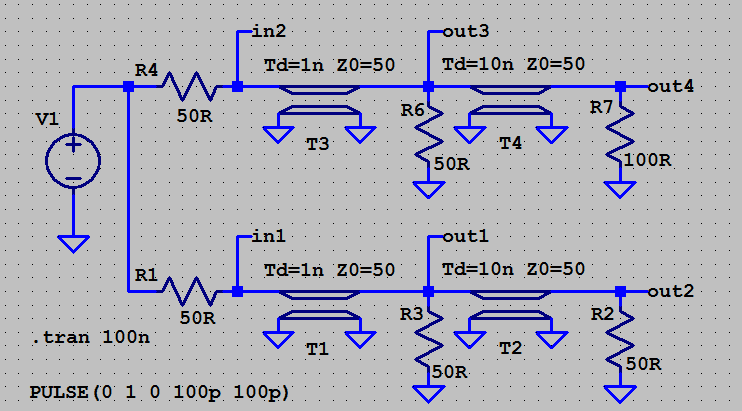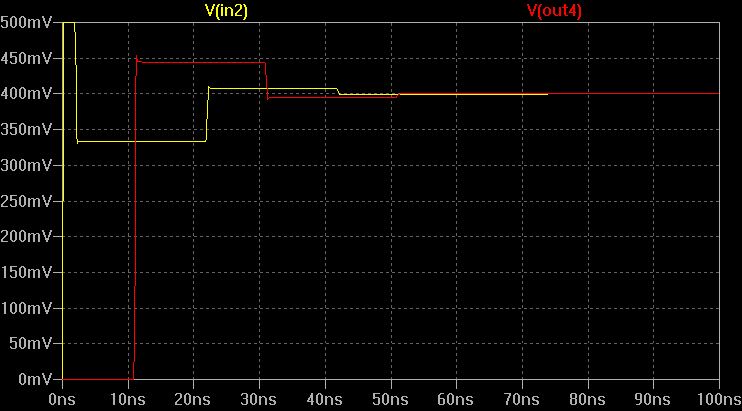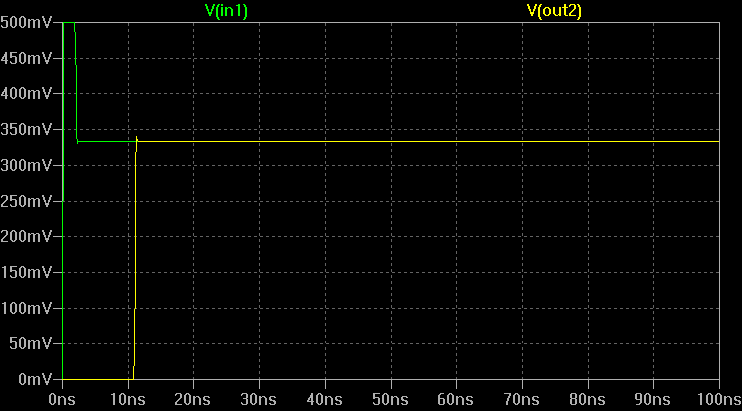# Transmission line with 2x termination

likephysics
Trying to work out the match for a transmission line with 2x termination.
Like the one here:
https://ibb.co/p4dY7pF
V1=1
R1=R2=T1=T2=50,
V1 sees R1 and T1. So it is divided to half.
Now you have 0.5V source with a 50 Ohms series impedance(T1) looking into a load that is a parallel combo of R3&T2, which is 25 Ohms.
So voltage at load is (0.5*25/75) = 0.16V.
This further reduces to a voltage source of 0.16v with 25 ohm series resistance and 50 ohm Tline?

(not an assignment, just trying to refresh by knowledge)

Mentor
I wouldn't call this a 2x terminated TL. It's a lot more complicated than that.

I would use the TL reflection coefficient equation applied over time for each transition, and maybe use Excel for a quick way to solve for the response to the transition. Do you have the simulation results for the waveform seen at the transmitter versus time?

(See the better reply below by Baluncore)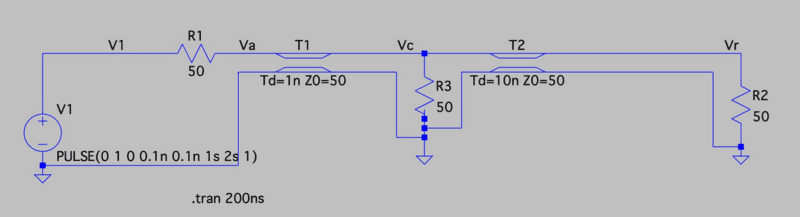Last edited:
A quick look suggests first simplify the circuit. Since T2 is terminated in a perfect match, T2 can be eliminated and the termination R2, moved to parallel R3, making 25 ohms. Now you have only one line, terminated in Zo/2.

The 1 volt step will appear as a half volt step at entry to T1.
2 ns later, the reflection from the 25 ohm termination will return to the correctly matched T1 input.

You then have a 50 ohm source resistor in series with a 25 ohm load.
So the steady state input to T1 will be 1/3 Vin, as will the output across R2.

••dlgoff, DaveE and berkeman
likephysics
I was trying to figure out the equivalent circuit starting from R3 to the load R2.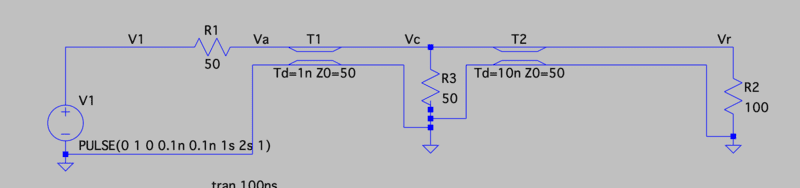Let's say R2=100 and T2=50, then the part from R3 would reduce to
Voltage source of 0.33V with series res of 25 ohm and a T line of 50 ohm?
The 25 doesn't make sense. Since R3=T2, this series res would be 0?
But then what if R3 is not equal to T2.

@berkemen, here's the sim result for ckt earlier.
https://ibb.co/mqmQMrg

I was trying to figure out the equivalent circuit starting from R3 to the load R2.
The input to T1 is series matched, so it will not return reflected energy from the T1:T2 junction.

When the terminal load R2 is changed from 50R to 100R, both ends of T2 become mismatched, which makes a resonator.

A transient on T2 will travel forwards and backwards until it settles at the terminal state, or is disturbed again by another transient.

The terminal state will be that of a voltage divider where ( 50R // 100R ) = 33R33; is driven through 50R.
33R33 / ( 33R33 + 50R ) = 0.400 Vin.